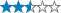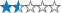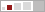# BC1 'Badges & Coins' Multi-cache

##### This cache has been archived.

Deceangi: Rather than keep this cache temporarily disabled (which should only be used for shortish periods) I'm archiving it. If/when the cache is ever replaced I'll gladly unarchive it assuming it still meets the guidelines.

Deceangi Volunteer UK Reviewer Geocaching.com

More
A cache by BarberClan
Hidden : 10/04/2006
Difficulty:Terrain:Size:(small)

#### Watch

How Geocaching WorksPlease note Use of geocaching.com services is subject to the terms and conditions in our disclaimer.

## Geocache Description:

The cache is in a screw-top cylinder covered in black tape.
The cache is quite small so recommended swaps would be... You guessed it, badges and coins!!!

To find this cache you will need to answer the questions below and complete a mathematical equation (you may need to bring a calculator).

The Questions
How much money would you be awarded if gave information leading to a conviction? Answer = A
In what year was the Allnatt Pavillion presented to Winnersh Council?
Answer = B (2 digit number for the day)
Answer = C (4 digit number for the year)
How many park benches are within the field? Answer = D
How many childrens play areas are in the field? Answer = E

The following questions relate to the play enclosure only.
How many swings are there? Answer = F
How many steps are there on the climbing frame? Answer = G
How many vertical chains are there on the bridge? Answer = H
How many bins are there in the play enclosure? Answer = I

The Cache
The following equation will provide the cache Northing:
(C/A)-(D(F+E)(B-H)/E)=X
X+D=Y
Y²+A=J

N51 25.J

The following equation will provide the cache Westing:
C-(D*A)²+3(G+E)=X
G(B+H)+(F²*E+I)=Y
X-Y=K
K+E=L

W000 L.0K

 A B C D E F G H I

Initial contents of this cache: